# Plus Two Computer Application Chapter Wise Questions and Answers Chapter 3 Functions

Students can Download Chapter 3 Functions Questions and Answers, Plus Two Computer Application Chapter Wise Questions and Answers helps you to revise the complete Kerala State Syllabus and score more marks in your examinations.

## Kerala Plus Two Computer Application Chapter Wise Questions and Answers Chapter 3 Functions

### Plus Two Computer Application Functions One Mark Questions and Answers

Question 1.
To read a single character for gender i.e. ’rri’ or ’f’.___function is used.
(a) getch()
(b) getchar()
(c) gets()
(d) getline()
(b) getchar()

Question 2.
To use getchar(), putchar(), gets() and puts(), which header file is used?
(a) iostream
(b) cstdio
(c) input
(d) output
(b) cstdioQuestion 3.
To use cin and cout, which header file is needed?
(a) iostream
(b) cstdio
(c) input
(d) output
(a) iostrem

Question 4.
Predict the output of the following code snippet
#include<cstdio>
int main()
{
for(int i=0; name[i]!=’\0′;i++)
putchar(name[i]);
}

Question 5.
From the following which is equivalent to the function getc(stdin)
(a) putchar()
(b) gets()
(c) getchar()
(d) puts()
(c) getchar()Question 6.
From the following which is equivalent to the function putc(ch,stdout)
(a) putchar(ch)
(b) ch=gets()
(c) ch=getchar()
(d) puts(ch)
(a) putchar(ch)

Question 7.
To print a single character at a time which function is used?
(a) puts()
(b) putchar()
(c) gets()
(d) getchar()
(b) putchar()

Question 8.
To read a string____function is used.
(?) puts()
(b) putchar()
(c) gets()
(d) getchar()
(b) gets()

Question 9.
To print a string_____function is used.
(a) puts()
(b) putchar()
(c) gets()
(d) getchar()
(b) puts()Question 10.
Consider the following code snippet
main()
{
char str;
gets(str);
for(int i=0,len=0;str[i]!-\0′;i++,len++);
cout<<“The length of the string is” <<len;
getch();
}
Select the equivalent for the under lined statement from the following
(a) int len= strlen(str)
(b) int len=strcmp(str)
(c) int len = strcount(str)
(d) None of these
(a) int len= strlen(str)

Question 11.
Arjun wants to read a string with spaces from the following which is suitable
(a) cin>>
(b) cin.getline(str,80)
(c) str=getc(stdin)
(d) none of these
(b) cin.getline(str,80)

Question 12.
State whether the following statement is true or false. The'<<‘ insertion operator stops reading a string when it encounters a space.
True.

Question 13.
The process of dividing big programs into small programs are called____.
Modularization.Question 14.
The big programs are divided into smaller programs. This smaller programs are called_____.
Functions.

Question 15.
The execution of the program begins at____function.
main function.

Question 16.
One of the following is not involved in the creation and usage of a user defined function
(a) Define a function
(b) Declare a function
(c) invoke a function
(d) None of these
(d) None of these

Question 17.
The default data type returned by a function is_____.
(a) float
(b) double
(c) int
(d) char
(c) int

Question 18.
After the execution of a function, it is returned back to the main function by executing____keyword.
return.

Question 19.
Supplying data to a function from the called function by using______.
parameters (arguments).

Question 20.
_____keyword is used to give a value back to the called function.
return.

Question 21.
____key word is used to specify a function returns nothing.
void

Question 22.
One of the following is not necessary in the function declaration. What is it?
(a) name of the function
(b) return type
(c) number and type of arguments
(d) name of the parameters
(d) name of the parameters.

Question 23.
A function declaration is also called_____.
prototype.Question 24.
Considerthe following declaration
int sum(int a , int b)
{
return a+b;
}
From the following which is the valid function call.
(a) n=sum(10)
(b) n=sum(10, 20)
(c) n=sum(10, 20, 30)
(d) n=sum()
(b) n=sum(10, 20)

Question 25.
The ability to access a variable or a function from some where in a program is called_____.
scope.

Question 26.
A variable ora function declared within a function is have_____scope.
local.

Question 27.
A variable or a function declared out side of all the functions is have_____scope.
global.

Question 28.
State True/False

1. A local variable exist till the end of the program
2. A global variable destroyed when the sub function terminates

1. False
2. FalseQuestion 29.
consider the following declaration
int x;
int main()
{

}
Here x is a____variable.
global.

Question 30.
consider the following declaration
int main()
{
int x;

}
Here x is a_____variable.
local.

Question 31.
______parameter is used when the function call does not supply a value for parameters.
default.

Question 32.
Consider the following function declaration with optional (default) arguments and state legal or illegal and give the reasons

1. int sum(int x=10, int y, int z)
2. int sum(int x=10, int y=20, int z)
3. int sum(int x=10, int y=20, int z=30)
4. int sum(int x, int y=20, int z)
5. int sum(int x, int y=20, int z=30)
6. int sum(int x, int y, int z=30)
7. int sum(int x=10, int y, int z=30)

There is a rule to make an argument as default argument,i.e., to set an argument with a value that must be in the order from right to left. All the arguments in the right side of an argument must be set first to make an argument as a default argument.

1. illegal, because y and z are not have values
2. illegal, because z has no value
3. legal
4. illegal, because z has no value
5. legal
6. legal
7. illegal, because x has a value but y has no value.

Question 33.
The parameter used to call a function is called_____.
actual parameter.Question 34.
The parameters appear in a function definition are_____.
formal parameters.

Question 35.
After the distribution of answer scripts, the teacher gives the Photostat copy of the mark list to the students to check the marks. If the students make any change that do not affect the original mark list. There is a similar situation to pass the arguments to a function. What is this method?
(a) call by value
(b) call by reference
(d) none of these
(a) call by value

Question 36.
Your class teacher gives you the original mark list to check the mark. If you make any change it will affect the original mark list. There is a similar situation to pass the arguments to a function. What is this method?
(a) call by value
(b) call by reference
(c) call by function
(d) none of these
(b) call by reference

Question 37.
Consider the following function declaration
int sum(int a, int b)
{
Body
}
Here the arguments are passed by______.
call by value method.Question 38.
Consider the following function declaration
int sum(int &a, int &b)
{
Body
}
Here the arguments are passed by______.
call by reference method.

Qn. 39
A function calls it self is known as______
recursive function.

Question 40.
Varun wants to copy a string by using strcpy() function. From the following which header file is used for this?
(a) cstdio
(b) cmath
(c) cstring
(d) cctype
(c) cstring

Question 41.
____is a named group of statements to perform a job /task and returns a value.
Function.

Question 42.
To use the function setw(), from the following which header file is used.
iomanip.h

Question 43.
In his C++ program Ajith wants to accept a lengthy text of more than one line. Which function in C++ can be used in this situation.
gets() function can be used to accept a lengthy text.

Question 44.
Choose the C++ function which can print a string.
(a) getche()
(b) putchar()
(c) getline()
(d) puts()
(d) puts()Question 45.
Which of the following is a console function?
(a) getline()
(b) write()
(c) put()
(d) getchar()
(d) getchar()

Question 46.
Pick the odd one out and give reason.
(a) abs()
(b) strlen()
(c) strcmp()
(d) strcpy()
(a) abs() – it is a mathematical function. All others are string functions.

Question 47.
Consider the following C++ statement and answer the following question:
char Word=”GOOD DAY”;
Identify the correct output statement to display the string
(a) write (word);
(b) cout.write(word);
(c) cout (word);
(d) cout.write (word, 10);
(d) cout.write(wond, 10);

Question 48.
When the number -7 is given as the argument of a predefined function in C++, it returns the value 7. Identify the function.
abs(): This function returns the absolute value.

Question 49.
Pick out the correct statement for prototype declaration from the following and also explain the various information it contains.
(a) product (int a, int b);
(b) int product (a,b);
(c) int product (int, int);
(d) product (int, int);
(c) int product(int, int);
This prototype specifies the return type, name of function, number, and type of arguments.Question 50.
One among the following function prototypes is wrongly written. Identify it. Also given reason.
(a) float test (float);
(b) float test (float, int);
(c) test (float);
(d) int test (int);
(c) test(float);
Here the prototype contains no return type.

Question 51.
A user defined function definition is given below. Choose the most appropriate function call statement from the options.
float calc(int x, float y)
{
return (x+y) / 2.0;
}
(a) calc (2, 4)
(b) calc (2.5, 4)
(c) calc (2.5, 4.5)
(d) calc (2, 4.5)
(d) calc(2, 4.5);

Question 52.
Which of the following statements are FALSE about a local function?
(a) Declared inside a function
(b) Accessible only within the function it is declared
(c) Accessible from anywhere in the program
(d) Declared outside all other functions
c and d are false to a local function.

### Plus Two Computer Application Functions Two Mark Questions and Answers

Question 1.
In a C++ program, you forgot to include the header file iostream.h. What are the possible errors occur in that Program? Explain ?
Proto type error. To use cin and cout the header file iostream is a must.

Question 2.
Pick the odd one out from the following and give reason.

• gets()
• getline()
• getch()
• getchar()

getline() – It is a stream function where as the others are console functions.Question 3.
Consider the following code snippet.
using namespace std;
int main()
{
int n;
cout<<“Enter a number”; cin>>n;
cout<<‘The number is “<<n;
}
Write down the names of the header files that must be included in this program
Here cin and cout are used so the header file iostream must be included.

Question 4.
How does C++ support modularity in programming
The process of converting big programs into smaller programs is known as modularisation. This small programs are called modules or sub programs or functions. C++ supports modularity in programming called functions.

Question 5.
The following assignment statement will generate a compilation error.
char str; str=”Computer”
Write a correct C++ statement to perform the same task
char str = “Computer”;

OR

char str;
strcpy(str,”Computer”); (The header file should be included).

Question 6.
float area(const float pi=3.1415, const float r)
{
r=10;
return pi*r;
}
Is there any problem? If yes what is it?
There is an error. The error is , Y is a constant T must be initialised and cannot be changed during execution.Question 7.
What are the jobs of a return statement in a program.
In the case of a sub function a return statement helps to terminate the sub function and return back to the main function or called function. But in the case of a main function it terminates the program.

Question 8.
Match the following

 (a) strcmp() (1) cctype (b) tolower() (2) cstring (c) sqrt() (3) cstdlib (d) abort () (4) cmath

(a) 2
(b) 1
(c) 4
(d) 3

Question 9.
How to invoke a function in C++ program.
A function can be called or invoked by providing the name of the function followed by the arguments in parenthesis Eg. sum(m,n);

Question 10.
Briefly explain constant arguments Constant arguments.
By using the key word const we can make argument (parameter) of a function as a constant argument.
The value of the const argument cannot be modified within the function.

Question 11.
void initialise()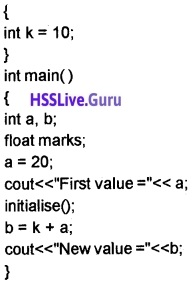1. Identify the error in the, above code and explain its reasons.
2. Correct the errors.

1. K is a local variable in the function initialize() – It is not accessible in main()
2. Making the variable K as global we can correct the error.Question 12.
List down the advantages of modular programming.
Merits of modular programming

• It reduces the size of the program
• Less chance of error occurrence
• Reduces programming complexity
• Improves reusability

Question 13.
Some statements are given below. Analyse these statements and predict the output:
char str1 (15], str2;
str1=” DATA”;
str2=” STORAGE”;
strcat (str2, str1);
cout<<str2;
The output is STORAGE DATA. The strings str2 and str1 are concatenated.

Question 14.
If char name [ ] = “Rajeev Kumar”; then what will be output of the following statement? cout<<strlen(name);
The length(number of characters) is 12 including space.

Question 15.
Choose the value of ‘n’ after executing the following statements in C++.
char s1[ ]=”KIRAN”; char s2[ ]=”kiran”;
int n = strcmp (s1,s2);
cout<<n;
(a) 0
(b) >0
(c) <0
(d) None of these
(c) <0. Here string 2, i.e. s2 is greaterthan string1 i.e. s1.
strcmp()- It is used to compare two strings and returns an integer.
Syntax: strcmp(string1 ,string2)

• if it is 0 both strings are equal.
• if it is greater than 0(i.e. +ve) string1 is greater than string2
• if it is less than 0(i.e. -ve) string2 is greater than string1Question 16.
C++ has a built-in function with which we get the result of 42.

1. Identify the name of the function.
2. Identify the header file for the above function.

1. pow(4, 2);
2. The header file used is cmath.

Question 17.
Consider the following C++ statements and predict the output.
int p=isalpha(‘5’);
cout<<p;
0.
isalpha() – To check whether a character is an alphabet or not. If the character is an alphabet it returns a value 1 otherwise it returns 0.

Question 18.
Predict the output of the following C++ statements:

1. cout<<toupper(‘a’);
2. cout<<(char) toupper(‘a’);

1. It prints 65
2. It prints AQuestion 19.
Differentiate formal arguments and actual arguments.
The parameter used to call a function is called actual parameter. The parameters appear in a function definition are formal parameters.

### Plus Two Computer Application Functions Three Mark Questions and Answers

Question 1.
Suresh wants to print his name and native place using a C++ program. The program should accept name and native place first
Name is: Suresh Kumar
# include<iostream>
# include<cstdio>
using namespace std;
int main()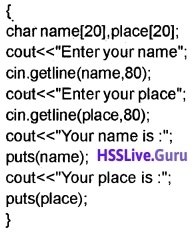Question 2.
“Programming is Fun”. Write a C++ program to read a string like this in lowercase and print it in UPPER CASE. Without using toupper() library function.
# include<iostream>
# include<cstdio>
using namespace std;
int main()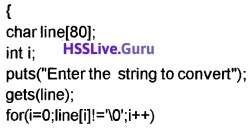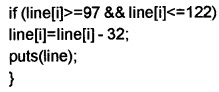Question 3.
An assignment, Kumar has written a C++ program which reads a line of text and print the number of vowels in it. What will be his program code?
# include<iostream>
# include<cstdio>
# include<cctype>
using namespace std;
int main()Question 4.
Write a program to display the following output
A
BB
CCC
# include<iostream>
using namespace std;
int main()Question 5.
Distinguish getchar and gets.
getchar is a character function but gets is a string function. The header file cstdio must be included. It reads a character from the keyboard.
Eg.
char ch;
ch=getchar();
cout<<ch;
gets is used to read a string from the key board. It reads the characters upto enter key. The header file
cstdio must be included.
char str;
cout<<” Enter a string”;
gets(str);

Question 6.
Write a program to check whether a string is palindrome or not. (A string is said to be palindrome if it is the same as the string constituted by reversing the characters of the original string. Eg. “MALAYALAM”, “MADAM”, “ARORA”, “DAD”, etc.
# include<iostream>
using namespace std;
int main()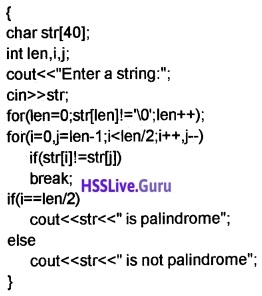Question 7.
Explain multi character function.
getline() and write() functions are multi character functions.
1. getline():
It reads a line of text that ends with a newline character. It reads white spaces also.
Eg.
char line;
cin.getline(line,80);

2. write():
It is used to display a string.
Eg.
charline;
dn.getline(line, 80);
cout.write(line, 80);

Question 8.
Distinguish between get() and put() functions.
1. get() function:
get() is an input function. It is used to read a single character and it does not ignore the white spaces and newline character.
Syntax is cin.get(variable);
Eg. char ch;
cin.get(ch);

2. put() function:
put() is an output function. It is used to print a character.
Syntax is cout.put(variable);
Eg. char ch;
cin.get(ch);
cout.put(ch);

Question 9.
Write a program to read a string and print the number of consonents
# include<iostream>
# include<cstdio>
# include<cctype>
using namespace std;
int main()Question 10.
Write a program to read a string and print the num¬ber of spaces.
# include<iostream>
# include<cstdio>
using namespace std;
int main()Question 11.
Write a program to count the number of words in a sentence
# include<iostream>
# include<cstdio>
using namespace std;
int main()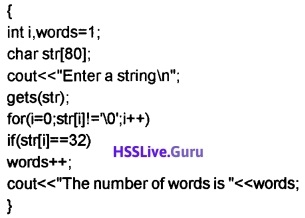Question 12.
Write a program to input a string and display its reversed string using console I / O functions only. For example if the input is “AND” the output should be “DNA”.
# include<iostream>
using namespace std;
int main()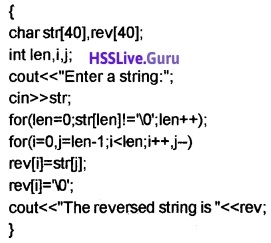Question 13.
Write a program to input a word(say COMPUTER) and create a triangle as follows.# include<iostream>
# include<cstring>
using namespace std;
int main()Question 14.
Write a program to input a line of text and display the first characters of each word. Use only console I /O functions. For example, if the input is “Save Water, Save Nature”, the output should be “SWSN”.
# include<iostream>
# include<cstdio>
using namespace std;
int main()Question 15.
Consider the following code snippet
char ch;
cout<< “Enter an alphabet”; cin>>ch;
cout<<toupper(ch);
What is the output of the above code? Give a sample output. If the above code is used in a computer that has no cctype file, how will you modify the code to get the same output?
It reads a character and convert it into uppercase.
Eg:
Enter an alphabet: a
The output is A.
If a computer has no cctype header file the code is as follows.
char ch;
cout<< “Enter an alphabet”; cin>>ch;
if (ch>=97 && ch<<122)
cout<

Question 16.
# include<iostream.h>
int main()
{
cout<<sum(2, 3);
}
int sum(int x, int y)
{return (x + y);}
On compilation on the program, an error will be dis-played. Identify and explain the reason. How can you rectify the problem
The compilation of the program starts from the first line and next line and so on( i.e. line by line). While compiling the line cout<<sum(2, 3); The compiler does not understand the word sum(2, 3) because it is not declared yet hence the error prototype required. To rectify this problem there are two methods
First method
Give the function definition just before the main function as follows.
# include<iostream>
using namespace std;
int sum(int x, int y)
{return (x+y);}
int main()
{
cout<<sum(2, 3);
}

Second Method
Give the function declaration(prototype only) in the main function as follows.
# include<iostream>
using namespace std;
int main()
{
int sum(int, int);
cout<<sum(2, 3);
}
int sum(int x, int y)
{return (x+y);}Question 17.
Considering the following function definition;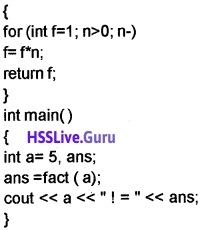The expected, desired output is 5! = 120
What will be the actual output of the program? It is not the same as above, why? What modification are required in the program to get the desired output.
The output is 0! = 120
Because the address of variable ‘a’ is given to the variable ‘n’ of the function fact(call by reference method). So the function changes its value (i.e. n- -) to 0. Hence the result.

To get the desired result call the function as call by value method in this method the copy of the value of the variable ‘a’ is given to the function. So the actual value of ‘a’ will not changed. So instead of int fact(int &n) just write int fact(int n), i.e., no need of & symbol.

Question 18.
A function is defined as follows
int sum (int a, int b=2)
{return (a+b);}
Check whether each of the following function calls is correct or wrong, Justify your answer

1. cout<<sum(2, 3);
2. cout<<sum( 2);
3. cout<<sum();

Here the function is declared with one optional argument. So the function call with minimum one argument is compulsory.

1. 0 It is valid. Here a becomes 2 and b becomes 3.
2. It is also valid . Here a becomes 2 and b takes the default value 2.
3. It is not a valid call. One argument is compulsory.

Question 19.
How do two functions exchange data between them? Compare the two methods of data transfer from calling function to called function.
There are two methods they are call by value and call by reference
1. call by value:
In call by value method, a copy of the actual parameters are passed to the formal parameters. If the function makes any change it will not affect the original value.

2. call by reference:
In call by reference method, the reference of the actual parameters are passed to the formal parameters. If the function makes any change it will affect the original value.Question 20.
Write down the operation performed by the following statements

1. int l=strten(“Computer Program”);
2. charch [] = tolower(“My School”);
3. cout<<(strcmp(“High”, “Low”)>0 ?

toupper(“High”):tolower(“Low”));

1. The built in function strlen find the length of the string i.e. 16 and assigns it to the variable I.
2. This is an error because tolower is a character function.
3. This is also an error because tolower and toupper are character functions.

Question 21.
A line of given length with a particular character is to be displayed. For example, ********** is a line with ten asterisks (*). Define a function to achieve this output
#include<iostream>
using namespace std;
void line (char ch, int n)Question 22.
int fib(int n)
{
if (n<3)
return 1;
else
return (fib(n-1) + fib(n-2));
}

1. What is the speciality of this function
2. How does it work ?
3. What will be the output of the following code?

for (int i=1; i<5; i++)
cout<<fib(i)<<‘\t’;
1. This function is a recursive function. That means the function calls itself.

2. It works as follows
if i = 1, The function fib calls with value 1. i.e. fib(1) returns 1
if i = 2, The function fib calls with value 2. i.e. fib(2) returns 1
if i = 3, The function fib calls with value 3. i.e. fib(3) returns fib(2) + fib(1) i.e. it calls the function again. So the result is 1 + 1 = 2
if i = 4, The function fib calls with value 4. i.e. fib(4) returns fib(3) + fib(2) i.e. it calls the function again. So the result is 2 + 1 = 3

3. The output will be as follows
1 1 2 3.Question 23.
Explain scope rules of functions and variables in a C++ program
1. Local variable or function:
A variable or function declared inside a function is called local variable or function. This cannot be accessed by the outside of the function.
Eg.
main()
{
int k;//local variable ,
cout<<sum(a,b); // local function
}

2. Global variable or function:
A variable or function declared out side of a function is called global variable or function. This can be accessed by any statements.
Eg.
int k; // global variable
int sum(inta, int b); //global function
main()
{
}

Question 24.
Briefly explain default arguments.
A default value can be set for a parameter(argument) of a function. When the user does not give a value the function will take the default value. An important thing remember is an argument cannot have a default value unless all arguments on its right side must have default value.

Functions with valid default arguments are given below

• float area(int x, int y, int z=30);
• float area(int x, int y=20, int z=30);
• float area(int x=10, int y=20, int z=30);

Functions with invalid default arguments are given below

• float area(int x=10, int y, int z);
• float area(intx, inty=20, int z);
• float area(int x=10, int y=20, int z);

Question 25.
Write a program to read a character and check whether it is alphabet or not. If it is an alphabet check whether it is upper case or lower case?
#include<iostream>
using namespace std;
int main()Question 26.
Write a program to read 2 strings and join them
# include<iostream>
# include<cstdio>
using namespace std;
int main()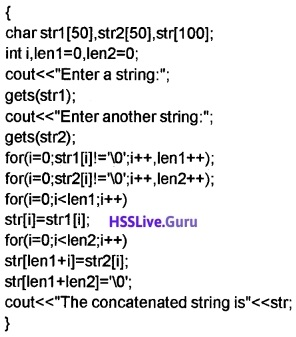Question 27.
Write a program to read 2 strings and compare it.
# include<iostream>
# include<cstdio>
# include<cstring>
using namespace std;
int main()Question 28.
Write a program to read a string and display the number of alphabets and digits and special characters.
# include<iostream>
# include<cstdio>
using namespace std;
int main()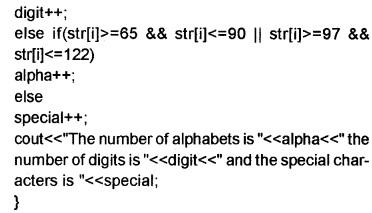Question 29.
A. void change(int&);
int main()B. void change(int);
int main()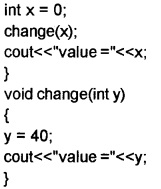1. Predict the output of both programs.

1. A. Output
value = 40
B. output
value = 0

2. In the first case (A) the argument x is passed by reference method. So the changes made in the function reflects in main()

In the second case (B) the argument x is passed by value method. So the changes made in the function will not reflect in main().

Question 30.
Write a program to read 2 strings and join them using string function
# include<iostream>
# include<cstring>
using namespace std;
int main()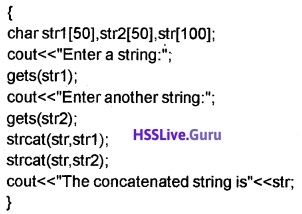Question 31.
Differentiate the outputs of the folloiwng C++ statements and also give reason

1. cout< <strcmp(“world”, “WORLD”);
2. cout<<strcmpi(“world”, “WORLD”);

1. >0 Here first string “world” is greater than “WORLD”.
2. It prints 0. Because strcmpi is same as strcmp() but it is not case sensitive. That means uppercase and lowercase are treated as same.

Question 32.
Match the following.

 1. strcmp() a. To combine two strings 2. strcpy () b. To get 5 from 25 3. strcat () c. To get 10 from -10 4. sqrt () d. To change a to A 5. abs() e. To compare two strings 6. toupper() f. To copy one string another

1-e, 2-f, 3-a, 4-b, 5-c, 6-d.

Question 33.
Write a C++ program to find the sum of first ‘N’ natural numbers using a user defined function.
# include<iostream>
using namespace std;
int sum(int n)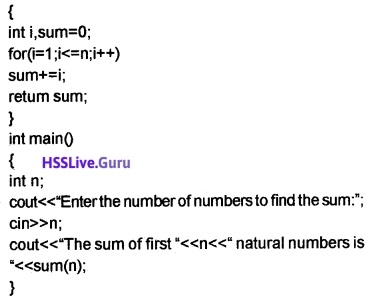Question 34.
Write the need for function prototype in a C++ program.
When the function is defined after the main function then there is an error called “function should have a prototype”. This is because of the function is defined after the main function. To resolve this a prototype should be declared inside the main function.

Question 35.
Write suitable function prototype after reading the following cases.

• Case I : The function Volume() takes two arguments, one is float the other is int and it returns its volume.
• Case II : A function Big() has no arguments and no return type.
• Case III: A function PrintO takes two floating point type arguments and nothing is returned.

• Case I: float Volume(float,int);
• Case II: void BigO; or void Big(void);
• Case III : void Print(float,float) or void Print(double,double);Question 36.
Find the error in the following C++ program and rectify it.
#include<iostream>
using namespace std;
int main ()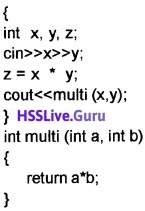Error 1: Here function prototype is missing.
Error 2: no need for variable z.
Error 3: no need of the statement z = x*y;
#include<iostream>
using namespace std;
int multi(int, int);
int main()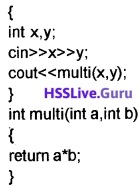Question 37.
Consider the following function definition.
int add (int a, int b=2, int c=5)
{
int s = a + b + c;
cout<<“Sum is :”<<s;
}
Predict the output of the above code forthe following function calls:

1. add(5, 8, 10). Here a = 5, b = 8 and c = 10. Then It prints 23(5 + 8 + 10)
2. add(5, 8). Here a = 5, b = 8 and no value for c then c will take the default value 5. Hence it prints 18(5 + 8 + 5(default value for c)).
3. add(5). Here a = 5 and no values for b and c, then b and c will take the default values 2 and 5 respectively. Hence it prints 12(5 + 2 + 5(default values for b and c)).

Question 38.
Consider the following C++ program, predict the output and justify it.
#include<iostream>
using namespace std;
intsqr(int&);
int main()The output are 25 and 6. Here the function uses call by reference method. The function call sqr(a) passes the original value to the function sqr. The function changes the value of b(here a and b are same) to 6. That means a also becomes 6.Question 39.
Differentiate local variable and global variable in C++ program.
1. Local scope:
A variable declared inside a block can be used only in the block. It cannot be used any other block.
Eg: int sum(int n1,int n2)
{
int s;
s=n1+n2;
return(s);
}
Here the variable s is declared inside the function sum and has local scope;

2. Global scope:
A variable declared outside of all blocks can be used anywhere in the program.
Eg:
int s;
intsum(int n1,int n2)
{
s=n1+n2;
return(s);
}
Here the variable s is declared out side of all functions and we can use variable s any where in the program.

Question 40.
Consider the following C++ code fragment and identify the local function and global function. Also justify your selection.
int main()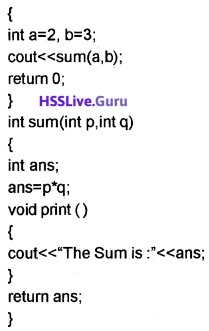Here the function print() is declared inside a function hence it is a local function but the function sum() is declared outside of all functions hence it is called global function.Question 41.
Read the following C++ program and identify the error and give reason.
# include<iostream>
using namespace std;
void disp(int);
int main()
int x=10; disp (x); return 0;The variable ‘x’ will not be printed because it is declared in the main() function. That is x is a local variable.

### Plus Two Computer Application Functions Five Mark Questions and Answers

Question 1.
What will be the output of the following code if the userenterthe value “GOOD MORNING”

1. char String ;
gets(string);
cout<<string;
2. char String ; cin>>string;
cout<<string;
3. char ch;
ch=getchar();
cout<<ch;
4. char String ;
cin.getline(string,9);
cout<<string;

1. GOOD MORNING
2. GOOD
3. G
4. GOOD MORNQuestion 2.
Read a string and print the number of vowels.
# include<iostream>
# include<cstdio>
# include<cctype>
using namespace std;
int main()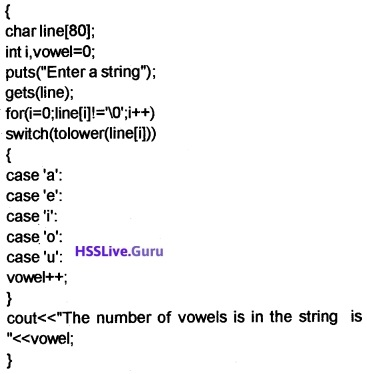Question 3.
Describe in detail about the unformatted console i/o functions.

1. Single character functions: This function is used to read or print a character at a time.
• getchar(): It reads a character from the keyboard and store it in a character variable.
Eg. char ch;
ch=getchar();
• putchar(): This function is used to print a character on the screen.
Eg. char ch;
ch=getcharO;
putchar(ch);
2. String functions: This function is used to read or print a string.
• gets(): This function is used to read a string from the keyboard and store it in a character variable.
Eg. char str;
gets(str);
• puts(): This function is used to display a string on the screen.
Eg. char str;
gets(str);
puts(str);Question 4.
Write a program to input a string and find the number of uppercase letters, lowercase letters, digits, special characters, and white spaces.
# include<iostream>
# include<cstdio>
using namespace std;
int main()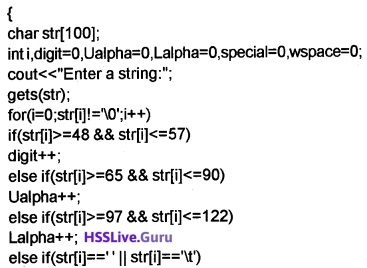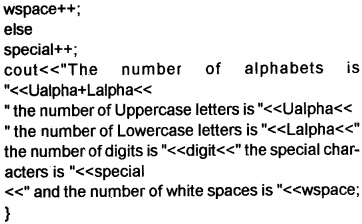Question 5.
Write a program to input a string and replace all lowercase vowels by the corresponding uppercase letters.
# include<iostream>
# include<cstdio>
using namespace std;
int main()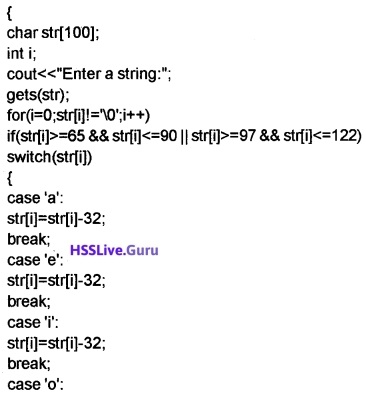Question 6.
A list of C++ built in functions are given. Classify them based on the usage and prepare a table with proper group names.

• strcmp()
• sin()
• getch()
• isalpha()
• pow()
• puts()
• strcat()
• tolower()
• getchar()
• isalnum()
• sqrt()
• exp()
• write()Question 7.
The factorial of a number, say N is the product of first N natural numbers. Thus, factorial of 5 can be obtained by taking the product of 5 and factorial of 4. Similarly factorial of 4 be found out by taking the product of 4 and factorial of 3. At last the factorial of 1 is 1 itself. Which technique is applicable to find the factorial of a number in this fashion? Write a C++ function to implement this technique. Also explain the working of the function by giving the number 5 as input.
A function calls itself is known as recursion.
# include<iostream>
using namespace std;
int fac(int);
int main()The working of this program is as follows. If the value of n is 5 then it calls the function as fac(5). The function returns value 5*fac(4). That means this function calls the function again and returns 5*4*fac(3). This process continues until the value n=1. So the result is 5*4*3*2*1 = 120.Question 8.
# include<iostream>
using namespace std;
int a = 0;
int main ()Write down the value displayed by the output of the above program with suitable explanation. What are the inferences drawn regarding the scope of variables?
The output is 061.
1. Global variable: A variable declared out side of all functions it is known as global variable.

2. Local variable: A variable declared inside of a function it is known as local variable.

If a variable declared inside a function(main or other) with the same name of a global variable. The function uses the value of local variable and does not use the value of the global variable.

Here int a=0 is a global variable. In the main function the global variable ‘a’ is used. There is no local vari-able so the value of ‘a’, 0 is displayed. The statement ‘a++’ makes the value of ‘a’ is 1. It calls the function showval with argument ‘a=1’.

The argument ‘x’ will get this value i.e. ‘x=T. But in the function showval there is a local variable ‘a’ its value is 5 is used. So this function returns 6 and it will be displayed. After this the value 1 of the global variable ‘a’ will be displayed. Hence the result 061.Question 9.
The following are function calling statements. Some of them will be executed, while some other generate compilation error. Write down your opinion on each of them with proper justification

1. char ch=getch();
2. sqrt(25);
3. strcat (“Computer”, “Program”);
4. double num = pow(2, 3, 5)
5. put char(getchar());

1. getch get a character from the console(key board) but does not echo to the screen. So we can’t read a character from the console.
2. It returns the square root of 25.
3. It concatenates Program to computer, i.e. we will get a string “computer program”
4. The function pow should contains only two arguments. But here it contains 3 arguments so it is an error. We can write this function as follows Double num = pow(pow(2, 3, 5)
5. It reads a character from the console and display it on the screen.

Question 10.
Short notes about character functions and string functions
a. Character functions:
1. isalnum(): It is used to check whether a character is alphabet or digit. It returns a non zero value if it is an alphabet or digit otherwise it returns zero.

2. isalpha(): It is used to check whether a character is alphabet or not. It returns a non zero value if it is an alphabet otherwise it returns zero.

3. isdigit(): It is used to check whether a character is digit or not. It returns a non zero value if it is digit otherwise it returns zero.

4. islower(): It is used to check whether a character is lower case alphabet or not. It returns a non zero value if it is a lower case alphabet otherwise it returns zero.

5. isupper(): It is used to check whether a character is upper case alphabet or not. It returns a non zero value if it is an upper case alphabet otherwise it returns zero.

6. tolower(): It is used to convert the alphabet into lowercase.

7. toupper(): It is used to convert the alphabet into upper case.

b. String functions:
1. strcpy(): This function is used to copy one string into another.

2. strcat(): This function is used to concatenate(join) second string into first string.

3. strlen(): This function is used to find the length of a string.

4. strcmp(): This function is used to compare 2 strings. If the first string is less than second string then it returns a negative value. If the first string is equal to the second string then it returns a value zero and if the first string is greater than the second string then it returns a positive value.Question 11.
Write a program to perform the following operations on a string

1. Length of a string
2. Search a character
3. Display the string

# include<iostream>
# include<cstdio>
using namespace std;
void len()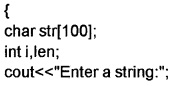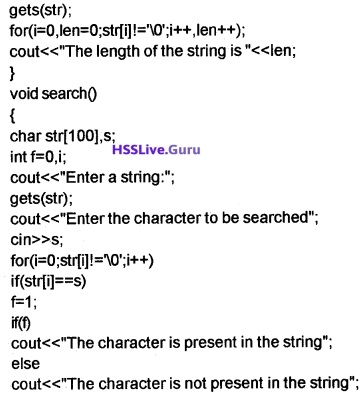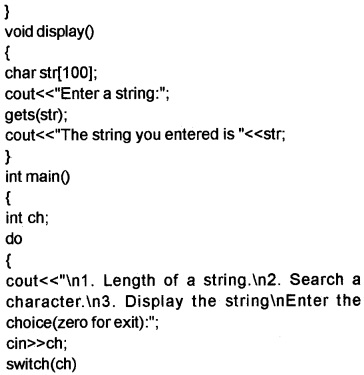Question 12.
Write functions to perform the following operations.

1. sqrt()
2. power of 2 numbers
3. sin
4. cos

# include<iostream>
# include<cmath>
using namespace std;
void sqroot()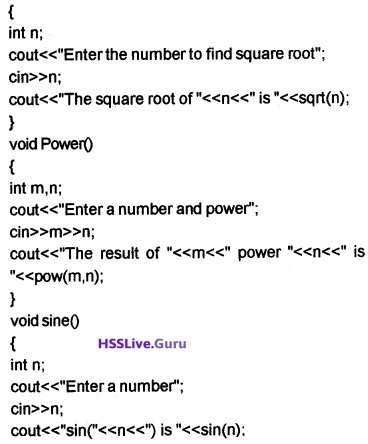Question 13.
Case I
# include<iostream>
using namespace std;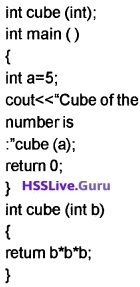Case II
# include<iostream>
using namespace std;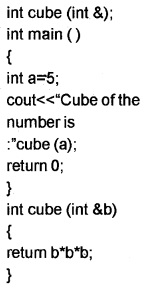1. Identify the type of function call in each case.
2. How do they differ?